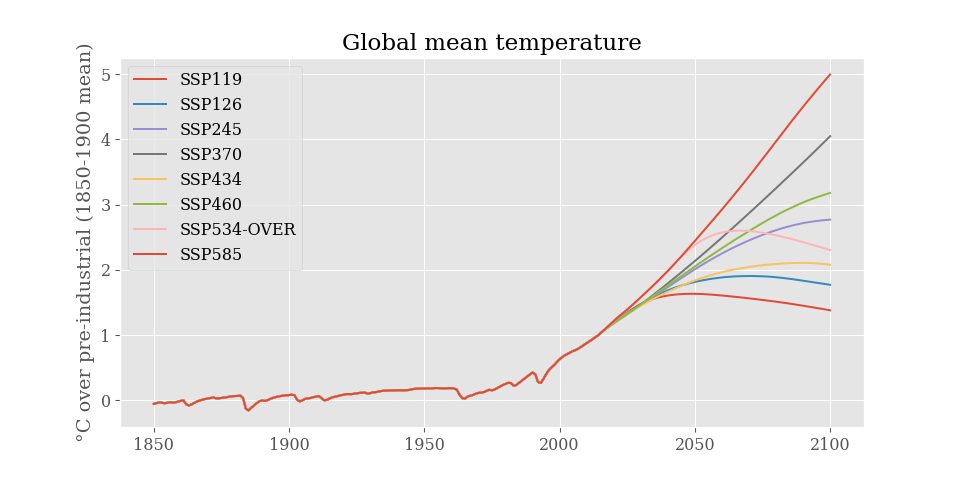# Usage¶

This repository also contains a Jupyter Notebook you can run live and experiment with, courtesy of the Binder project. The notebook can be viewed as a static version using nbviewer.

## Basic example¶

```import pyhector

output = pyhector.run(pyhector.rcp26)
```

```import pyhector
from pyhector import rcp26, rcp45, rcp60, rcp85

import matplotlib.pyplot as plt

for rcp in [rcp26, rcp45, rcp60, rcp85]:
output = pyhector.run(rcp, {"core": {"endDate": 2100}})
temp = output["temperature.Tgav"]
# Adjust to 1850 - 1900 reference period
temp = temp.loc[1850:] - temp.loc[1850:1900].mean()
temp.plot(label=rcp.name.split("_"))
plt.title("Global mean temperature")
plt.ylabel("°C over pre-industrial (1850-1900 mean)")
plt.legend(loc="best")
plt.show()
```